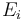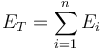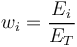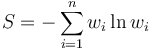# Shannon Index

## Definition

For the purpose of measuring name or sector concentration, the Shannon Index (also entropy index) is defined as the sum product of relative portfolio shares of the exposures, times the natural logarithm of the exposures.

## Details

More precisely, if we have n exposures$E_i$ summing up to a total exposure of$E_T = \sum^{n}_{i=1} E_{i}$

where each exposure fraction is defined as$w_{i} = \frac{E_i}{E_T}$

then the Shannon index is defined as$S = - \sum^{n}_{i=1} w_{i} \ln{w_{i}}$

None

None

None

## Implementations

Open Source implementations of the Shannon index are available in